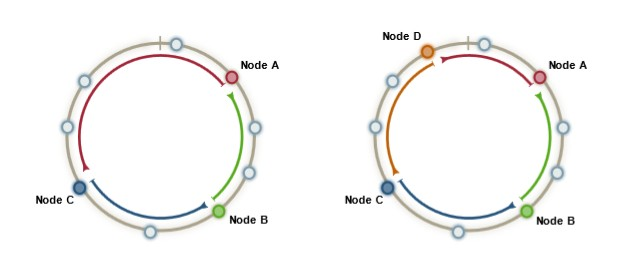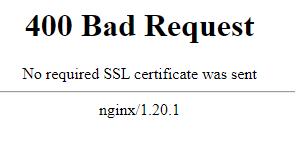# Collisions of Hash or Identifier Generation

A collision happens when a function produces the same result while it should be unique.

## Calculation

All calculation of collisions are based on the birthday problem 1).

The birthday problem tries to find the chance of two persons having:

• the same birthday (over a set of 365 days)
• in a classroom (over a set of N classmate)

If you translate:

• the same birthday by a collision
• the number of day in a year by the possible number of generated hash or id (m)
• the number of classmate by the number of generated values (n)

If $n=m$ , the probability is 100% (ie if you have 365 students, you are sure to have two students with the same birthday)

Applied to a collision problem, if we use the square approximation 2) (works well for probability under $\frac{1}{2}$ ), we get the number of value to generate n to have the probability p(n) is: $$n \approx \sqrt{2m \times p(n)} \approx \sqrt{2 \times \text{number of possible generated values} \times p(n)}$$ If we create a random identifier of length N in a set of k possible characters, m can be calculated with the permutation formula $$m = N^k$$ The applied formula is then: $$n \approx \sqrt{2 \times N^k \times p(n)}$$ or for the length of the identifier k (after a little bit of math) $$k = log_N(\frac{n^2}{2 \times p(n)})$$

The below forms applied this formula and calculates the identifier length needed for a random generated identifier

## Type

### Hash

An hash collision happens when two different inputs produce the same hash result.

It may happen because the hash is just a number in a possible range of value. A good hash function should generate in this range values that should be generated uniformly (ie almost randomly)

You can use the previous collision probability formula for any hash.

For instance, for for the md5,

Below is a table of the odds of a hash collision when you know the number of value to hash 3). You get for sure a collision with a probability of 100% (1 on 1)

You can read the below diagram as:

• for a hash with a length of 64-bit
• you need 192 million input
• to have a chance of 1 / 1000 to get a collision (four-of-kind in poker)

### Id

An id collision happens when the random identifier generator produce the same id twice.

See:

## Resolution

If the hash value is used to store data, the duplicate data can be stored at the same location and a lookup should be performed on a real unique identifier. Hash_table Collision_resolution

Recommended PagesCryptography - Hash

A hash function is an encryption crypto algorithm that takes as data as input (possibly large and of variable-sized) and produces a short fixed-length integer value (generally printed as an hexadecimal...Function - MD5 Hash function (Message Digest 5)

MD5 (Message Digest 5) is a hash function The primary function of an MD5 algorithm is to generate a message digest value (128-bit cryptographic), hence the name. md5 is not a secure hash algorithm....How to configure certification based client authentication with Nginx ?

This article shows you how to configure a client authentication via the ownership of a certificat on a Nginx web server. The server should be already configured for HTTPS as client certificate (client...JSON - ObjectId

ObjectId are generated identifier (known as surrogate) with the intent to be unique for a Json. ObjectId are custom UUID that are created from: a counter timestamp (milliseconds) node id (IP...Logical Data Modeling - (Surrogate | Substitute | Synthetic | Generated ) Primary key

A surrogate key is a substitute primary key for when: the data entity are created in distributed way you don't have access to a central entity such as database to create a simple sequence you don't...
What is a UUID - Universally Unique IDentifier - (also known as GUID) ?

UUID (Universally or Global Unique IDentifier) are generated identifier that are guaranteed to be unique and avoid collision